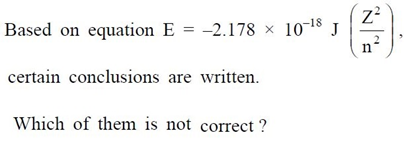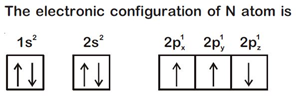# Structure of Atom NEET Questions : NEET Previous year questions

1. The number of protons, neutrons and electrons in 175Lu71, respectively, are

(NEET 2020)

(a) 71, 104 and 71                     (b) 104, 71 and 71

(c) 71, 71 and 104                     (d) 175, 104 and 71

Chemistry Notes

Physics Notes

Biology Notes

2. Be2+ is isoelectronic with which of the following ions?                      (2014)

(a) H+                 (b) Li+2+

(c) Na,               (d) Mg

3. Isoelectronic species are                                                           (2000)

(a) CO, CN, NO+, C 2–

(b) CO, CN, NO, C2 –2

(c) CO+, CN+, NO, C2

(d) CO, CN, NO, C2

6. Which of the following series of transitions in the spectrum of hydrogen atom falls in visible region?                                                                   (NEET 2019)

(a) Brackett series            (b) Lyman series

(c) Ballmer series             (d) Panchen series

7. Calculate the energy in joule corresponding to light of wavelength 45 nm.

(Planck’s constant, h = 6.63 × 10–34 J s, speed of light, c = 3 × 108 m s –1) (2014)

(a) 6.67 × 1015                (b) 6.67 × 1011

(c) 4.42 × 10–15                      (d) 4.42 × 10–18

8. The value of Planck’s constant is 6.63 × 10–34 Js. The speed of light is 3 × 1017 nm s–1. Which value is closest to the wavelength in nanometer of a quantum of light with frequency of 6 × 1015 s–1?                                   (NEET 2013)

(a) 50                      (b) 75

(c) 10                      (d) 25

9. According to law of photochemical equivalence the energy absorbed (in ergs/mole) is given as (h = 6.62 × 10–27 ergs, c = 3 × 1010 cm s–1,

NA = 6.02 × 1023 mol–1)                                          (NEET 2013)

(a) 1.196 x 108 / λ                      (b) 2.859 x 105 / λ

(c) 2.859 x 1016 / λ                    (d) 1.196 x 1016 / λ

10. The energies E1 and E2 of two radiations are 25 eV and 50 eV respectively. The relation between their wavelengths i.e., λ1 and λ2 will be                     (2011)

(a) λ1 = λ2                        (b) λ1 = 2 λ2

(c) λ1 = 4 λ2                      (d) λ1 = ½ λ2

14.(NEET 2013)

(a) Equation can be used to calculate the change in energy when the electron
changes orbit.

(b) For n = 1, the electron has a more negative energy than it does for n = 6 which
means that the electron is more loosely bound in the smallest allowed orbit.

(c) The negative sign in equation simply means that the energy of electron bound to
the nucleus is lower than it would be if the electrons were at n4 n3 the
infinite distance from the nucleus.

(d) Larger the value of n, the larger is the orbit radius.

15. According to the Bohr Theory, which of the following transitions in the hydrogen atom will give rise to the least energetic photon?         (Mains 2011)

(a) n = 6 to n = 1               (b) n = 5 to n = 4

(c) n = 6 to n = 5                (d) n = 5 to n = 3

16. The energy of second Bohr orbit of the hydrogen atom is –328 kJ mol–1; hence the energy of fourth Bohr orbit would be                                 (2005)

(a) – 41 kJ mol–1                (b) –82 kJ mol–1

(c) –164 kJ mol–1              (d) –1312 kJ mol–1

26. In hydrogen atom, the de Broglie wavelength of an electron in the second Bohr orbit is [Given that Bohr radius, a0 = 52.9 pm]                           (NEET 2019)

(a) 211.6 pm                (b) 211.6 π pm

(c) 52.9 π pm               (d) 105.8 pm

27. A 0.66 kg ball is moving with a speed of 100 m/s. The associated wavelength will be                                                                                 (Mains 2010)

(h = 6.6 × 10–34 J s)

(a) 6.6 × 10–32 m               (b) 6.6 × 10–34 m

(c) 1.0 × 10–35 m               (d) 1.0 × 10–32 m

29. The measurement of the electron position is associated with an uncertainty in momentum, which is equal to 1 × 10–18 g cm s–1. The uncertainty   (2008)

in electron velocity is (mass of an electron is 9 × 10–28 g)

(a) 1 × 105 cm s–1            (b) 1 × 1011 cm s–1

(c) 1 × 109 cm s–1                  (d) 1 × 106 cm s–1

30. Given : The mass of electron is 9.11 × 10–31 kg, Planck constant is 6.626 × 10–34 J s, the uncertainty involved in the measurement of velocity within a (2006)

distance of 0.1 Å is

(a) 5.79 × 105 m s –1 (b) 5.79 × 106 m s –1

(c) 5.79 × 107 m s –1 (d) 5.79 × 108 m s –1

36. 4d, 5p, 5f and 6p orbitals are arranged in the order of decreasing energy. The correct option is                                                                           (NEET 2019)

(a) 5f > 6p > 4d > 5p             (b) 5f > 6p > 5p > 4d

(c) 6p > 5f > 5p > 4d             (d) 6p > 5f > 4d > 5p

37. Orbital having 3 angular nodes and 3 total nodes is               (NEET 2019)

(a) 5p           (b) 3d           (c) 4f           (d) 6d

38. Which one is a wrong statement?                       (NEET 2018)

(a) Total orbital angular momentum of electron in s-orbital is equal to zero.

(b) An orbital is designated by three quantum numbers while an electron in an atom
is designated by four quantum numbers.

(c)(d) The value of m for dz2 is zero.

39. Which one is the wrong statement?                                        (NEET 2017)

(a) The uncertainty principle is ΔE x Δt ≥ h/4 π

(b) Half filled and fully filled orbitals have greater stability due to greater exchange energy, greater symmetry and more balanced arrangement.

(c) The energy of 2s-orbital is less than the energy of 2p-orbital in case of hydrogen like atoms.

(d) de-Broglie’s wavelength is given by λ = h/mv, where m = mass of the particle, v = group velocity of the particle.

40. How many electrons can fit in the orbital for which n = 3 and l = 1? (2016)

(a) 2                                           (b) 6

(c) 10                                         (d) 14

42. Two electrons occupying the same orbital are distinguished by           (2016)

(a) Azimuthal quantum number

(b) Spin quantum number

(c) Principal quantum number

(d) Magnetic quantum number

43. Which is the correct order of increasing energy of the listed orbitals in the atom of titanium?                                                                                       (2015)

(At. no. Z = 22)

(a) 4s 3s 3p 3d             (b) 3s 3p 3d 4s

(c) 3s 3p 4s 3d              (d) 3s 4s 3p 3d

44. The number of d-electrons in Fe2+ (Z = 26) is not equal to the number of electrons in which one of the following?                                 (2015)

(a) d-electrons in Fe (Z = 26)

(b) p-electrons in Ne (Z = 10)

(c) s-electrons in Mg (Z = 12)

(d) p-electrons in Cl (Z = 17)

45. The angular momentum of electron in ‘d’ orbital is equal to (2015)

(a) 2√3h  (b) 0h     (c) √6h (d) √2h

46. What is the maximum number of orbitals that can be identified with the following quantum numbers? n = 3, l = 1, ml = 0                        (2014)

(a) 1              (b) 2

(c) 3              (d) 4